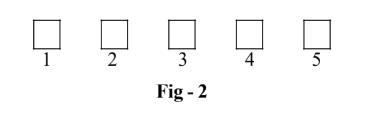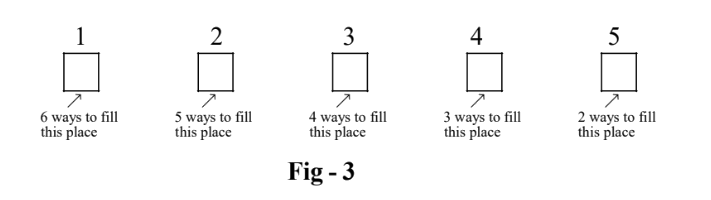# Fundamental Principle of Counting

Go back to  'P & C, Binomial Theorem'

# P & C, Binomial

This chapter is one of the most interesting chapters that we’ll study at this level. The beauty and challenge of this branch of mathematics lies in the innumerous tricks and mathematical artifices that abound in this subject. Clarity of thought, more than anything else, is what is required to understand the subject properly. Also, you’d do best if you refrain from memorising any formulae or particular cases here; concentrate on building a logical approach, solving everything from first principles.

The main objective of this chapter is to count. Given a set U of things or objects or persons ( or whatever), we need to arrange a subset S of U (according to some constraints) or select a subset S of U (again, according to certain criteria). In fact, we are actually interested in counting the number of such arrangements or selections. Read the following questions:

“From a team of 15 cricket players, how can we select a playing team of 11players?

“There are 20 people whom we need to seat in 2 rows of 10 seats each. How many ways exist of doing so?

“From a deck of 52 playing cards, in how many ways can we select two red cards and three black cards?”

“How many rectangles exist on a standard 8×8 chessboard?”

“How many factors does 144000 have? In general, how many factors does a natural number N have?”

These are some of the many types of questions which we’ll learn to solve in this chapter.

We’ll build a systematic approach to deal with such counting issues. To really appreciate the beauty of the solving techniques that we’ll develop, you are urged to try out each and every question that we solve here, on your own first, and only then look at the solution. Only this approach will help you solve counting questions elegantly.

# FUNDAMENTAL PRINCIPLE OF COUNTING

The fundamental principle of counting is so fundamental that you already must have used it practically a lot many times without realising it. In other words, this principle is already programmed into our minds. A logical,  step-by-step application of this principle gives rise to the entire subject of permutations and combinations.

Suppose you have 4 boys and 3 girls. From this group of 7, you want to select a couple ( a boy and a girl). How many ways exist of forming this couple?

Let us label the boys as $${B_1},\,\,{B_2},\,\,{B_3}\;\;{\text { and }} \;{B_4}$$  and the girls as $${G_1},\,\,{G_2},\;{\text { and }}\;{G_3}$$ .

There are 4 ways to choose a boy. Once you’ve chosen a boy, say $${B_2}$$ , there are now 3 ways to choose a girl. In other words, the boy $${B_2}$$ can form the couple $$({B_2}\,\,{G_1}),\,\,({B_2}\,\,{G_2})\,\,\,{\rm{or }}({B_2}\,\,{G_3})$$ . Similarly, for every other boy, there exist 3 girls with whom that boy can be paired. Thus, the total number of ways in which a pair can be formed is:

\begin{align}&N = \left( {{\text{No}}{\text {. of ways of selecting a boy}}} \right) \times \left( {{\text {No}}{\text {. of ways of selecting a girl}}} \right)\\&\,\,\,\,\,\, = 4 \times 3\\&\,\,\,\,\,\, = 12\end{align}

The most crucial aspect in this calculation is that you must realise that the task of selecting a boy is independent of the task of selecting a girl. This means that which boy you select has no effect what so ever on which girl you select; the selection of a boy and that of a girl are independent ofeach other.

Another subtle point must be made here. There are 4 ways to select a boy. These 4 ways are mutually exclusive. This means that a selection of any particular boy, once made, rules out the selection of the other 3 boys. Similarly, there are 3 mutually exclusive ways to select a girl.

Let us consider another example now. We need to travel from New Delhi, India to Fiji Islands (in the South Pacific Ocean). We must change flights, first at Singapore and then at Sydney, Australia. There are 6 different flights available from New Delhi to Singapore, 5 from Singapore to Sydney and 3 from Sydney to Fiji.How may ways exist of making a flight plan from New Delhi to Fiji?

Assume that we are in Singapore. From Singapore, we have 5 available flights to Sydney (5 mutually exclusive ways). For each of these 5 flights, we have 3 further ways (flights) from Sydney to Fiji. Thus, we have a total of 5 × 3 = 15 ways of travelling from Singapore to Fiji.

Now, how many ways do we have to reach Singapore from New Delhi in the first place? 6 flights. For each of these 6 flights, we have 15 further ways of reaching Fiji From Singapore (as we just calculated in the preceding paragraph). Thus, the total number of ways N of travelling from New Delhi to Fiji is 6 × 15 = 90. In other words,

$N = \left( \begin{array}{l}{\rm{No}}{\rm{. of \;flights\; from\; New\; }}\\{\rm{Delhi \;to\; Singapore}}\end{array} \right) \times \left( \begin{array}{l}{\rm{No}}{\rm{. of \;flights \;from\; }}\\{\rm{Singapore\; to\; Sydney}}\end{array} \right) \times \left( \begin{array}{l}{\rm{No}}{\rm{.of\; flights\; from \;}}\\{\rm{Sydney\; to Fiji}}\end{array} \right)$

Intuitively easy, isn’t it?

Let us now generalise the results of these two examples into our fundamental principle of counting.

Consider the set of tasks  $$\{ {T_1},\,\,{T_2},\,\,{T_3}\,\,...\,\,{T_n}\}$$ which are all independent of each other. This means that task $${T_i}$$    has “no relation ” to task  $${T_j}{\text { if }}\;i \ne j;$$  the choice of how to accomplish task $${T_i}$$  has thus “no effect” on the choice of how to accomplish talk $${T_j}\;\text{for}\;i \ne j$$  for , (you’ll realise the meaning of “no relation” and “no effect” more  specifically later). Task  $${T_i}$$ can be accomplished in  $${k_i}$$ mutually exclusive ways.

The fundamental principle of counting says that the set of tasks $$\{ {T_1},\,\,{T_2},\,\,{T_3}\,\,...\,\,{T_n}\}$$ can be accomplished in  $${k_1} \times {k_2} \times {k_3} \times ... \times {k_n}$$  ways.

Here are more examples that illustrate this simple yet extremely powerful principle. A proper understanding of this principle is absolutely essential for the subject of counting to be fully comprehensible.

•         Think of a standard six-faced die, with the markings 1, 2, 3, 4, 5 and 6. Suppose that you have two such dice. When these two dice are thrown call the number that shows up on the first die x and the one on  the second die y. How many pairs $$(x,\,\,y)$$  are possible?

There are  6 mutually exclusive ways in which a number can show up on the first die. Similarly, 6 such ways exist for the second die. The fundamental principle of counting says that the total number of possible pairs is 6 × 6 = 36.

•      Suppose that we have a (unlimited) supply of the letters $$A,\,\,B,\,\,C,\,\,D,\,\,E,\,\,F$$  available with us. How many 5-letter chains can we form using these letters?

Imagine 5 blank spaces for the 5-letter chain that we are supposed to form (numbered 1 to 5):•  We now have 5 tasks at hand; each task correspond to filling a space in Fig. 1. Realise that these 5 tasks are independent of each other. For example, what you fill in place 2 has no effect on what you fill in place 5 since   you’ ve been assured an unlimited supply of letters. Each task can be accomplished in 6 possible ways. For example, you can fill place -1 in 6 possible ways: with $$A,\,\,B,\,\,C,\,\,D,\,\,E,\,\,{\rm{or}}\,\,F$$ . Thus, by the fundamental principle of counting, the total number of ways to form the 5-letter chain would be $$6 \times 6 \times 6 \times 6 \times 6 = {6^5}$$ .

• Now consider the scenario when you don’t have an unlimited supply of letters available. Suppose that you have only one of each of the 5 letters available. How many 5-letter chains can be formed now?

You must realize that this limited-supply-of-letters situation is very different from the previous one. In this situation, once you fill a particular place with a particular letter, you are left with one letter less to choose from, from the remaining places.

Let us start with filling the places from left to right. To fill place -1, we have 6 letters to choose from, but once we’ve filled place-1 , we now have only 5 letters to choose from, to fill place-2. Continuing in this way, place-3, place - 4 and place -5 can then be filled in 4, 3 and 2 ways respectively.The fundamental principle of counting tells us that the total number of chains in this case will be $$6 \times 5 \times 4 \times 3 \times 2 = 720$$  .You might think that in this case filling up place-i and place-j are not independent tasks. In other words, how we fill place-i has a certain relation to how we fill place-j . For example, if we fill place-1 with A, we cannot fill any other place with A and thus, how we fill place -1 has a definite effect on how we are able to fill the other places. This is definitely true. But when we talk about the independence of two events, it is in a different sense:

Let event X be filling place-1 and event Y be filling place-2 after event X has been accomplished, i.e. after place-1 has been filled. There are 6 ways of accomplishing X and 5 ways of accomplishing Y. Which 5 letters contribute to event Y (i.e which of the 5 remaining letters can we choose for place -2) definitely depends on how event X was accomplished, but the event Y is itself the choice of one of the 5 symbols contributing to event Y. The number of ways in which this choice can be made is still independent of how event X was accomplished. Whatever selection was made for event X, the number of ways in which Y can be accomplished still remains 5. This is the sense that should be attached to the phrase “independent events”.

These three examples should have given you a pretty good idea about three concepts : mutually exclusive events, independent events and the fundamental principle of counting. Ponder over these examples for a bit longer and think up examples of you own till your feel very comfortable with these new concepts.

Learn from the best math teachers and top your exams

• Live one on one classroom and doubt clearing
• Practice worksheets in and after class for conceptual clarity
• Personalized curriculum to keep up with school